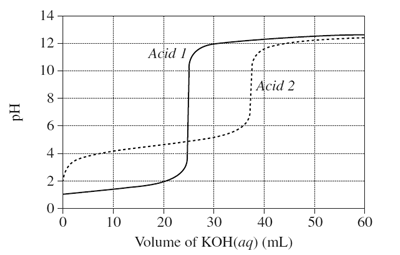Acid base (2009 NSW HSC) 1) The graph shows changes in pH for the titrations of equal volumes of solutions of two monoprotic acids, Acid 1 and Acid 2.(a) Explain the differences between Acid 1 and Acid 2 in terms of their relative strengths and concentrations. Solution (b) Name the salt produced by the reaction of an acid of the same type as Acid 2 with KOH(aq). Solution (c) Calculate the concentration of hydrogen ions when 20 mL of KOH(aq) has been added to Acid 1. Solution (d) Why would phenolphthalein be a suitable indicator for both titrations? Solution